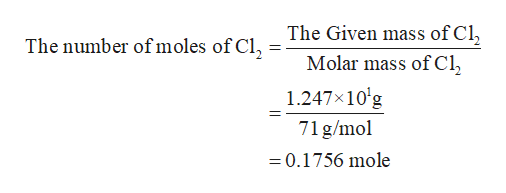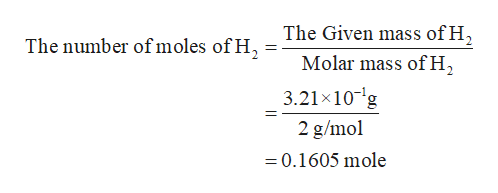# In the following chemical reaction between H​​ and Cl​​ to produce HCl, what is the sum of the mass of HCl produced plus the mass of left over reactants when 3.21E-1 g of H​​ completely reacts with 1.247E+1 g of Cl​​? H​​(g) + Cl​​(g) → 2HCl(g)

Question
59 views

In the following chemical reaction between H​​ and Cl​​ to produce HCl, what is the sum of the mass of HCl produced plus the mass of left over reactants when 3.21E-1 g of H​​ completely reacts with 1.247E+1 g of Cl​​? H​​(g) + Cl​​(g) → 2HCl(g)

check_circle

Step 1

The molar masses for Cl2(g), H2(g), and HCl(g) are 71 g/mol, 2 g/mol, and 36.5 g/mol respectively. The given masses for Cl2(g) and H2(g) are 1.247×10+1 g and 3.21×10-1 g respectively.

Step 2

The number of moles in the given masses of Cl2(g) is calculated as shown below. The number of moles in the given masses of Cl2(g) is 0.1756 moles.help_outlineImage TranscriptioncloseThe Given mass of Cl Molar mass of Cl The number of moles of Cl, 1.247x10'g 71 g/mol =0.1756 mole fullscreen
Step 3

The number of moles in the given masses of Cl2(g) is calculated as shown below. The nu...help_outlineImage TranscriptioncloseThe Given mass of H The number ofmoles of H Molar mass ofH2 3.21 x10 g 2 g/mol =0.1605 mole fullscreen

### Want to see the full answer?

See Solution

#### Want to see this answer and more?

Solutions are written by subject experts who are available 24/7. Questions are typically answered within 1 hour.*

See Solution
*Response times may vary by subject and question.
Tagged in

### States of matter